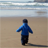# New to Qlik Sense

If you’re new to Qlik Sense, start with this Discussion Board and get up-to-speed quickly.

Announcements
Read about the latest Qlik Community enhancements on the Community News blog!
cancel
Showing results for
Did you mean:Contributor II

## Custom Sort with Alphanumeric values

Dear Fellows,

I am struggling to order some alphanumeric values that indicate tiers of customer spend. I want them to appear in the following order in a horizontal bar chart:

< zero
zero
>= 0 < 1,000
>= 1,000 < 5,000
>= 5,000 < 10,000
>= 10,000 < 20,000
>= 20,000 < 50,000
>= 50,000 < 100,000
>= 100,000 < 250,000
>= 250,000 < 500,000
nulls

I am using this Match expression in the sorting area:

Match(SpendTotal,'< zero','zero','>= 0 < 1,000','>= 1,000 < 5,000','>= 5,000 < 10,000','>= 10,000 < 20,000','>= 20,000 < 50,000','>= 50,000 < 100,000','>= 100,000 < 250,000','>= 250,000 < 500,000')

But when I choose Ascending I get these results:

zero
>= 5,000 < 10,000
>= 1,000 < 5,000
>= 0 < 1,000
< zero
>= 100,000 < 250,000
>= 10,000 < 20,000
>= 20,000 < 50,000
>= 50,000 < 100,000
>= 250,000 < 500,000
nulls

And with Descending I get these results:

>= 250,000 < 500,000
>= 5,000 < 10,000
>= 1,000 < 5,000
>= 0 < 1,000
< zero
>= 100,000 < 250,000
>= 10,000 < 20,000
>= 20,000 < 50,000
>= 50,000 < 100,000
zero
nulls

I guess it has something to do with the >= symbols and initial string value but I do not know how to overcome this.

I would be very grateful for any suggestions.

Kindest regards

Simon

Labels (4)

• ### Sorting of dimensions

1 Solution

Accepted SolutionsContributor II
Author

I eventually resolved this using the Sort Cards in the Data Manager.

3 RepliesPartner

Hi ,

Try with dual function. check out this post

instead of creating a sort order field u can directly define the sorting order while creating the buckets .

BR,

SaurabhContributor II
Author

Thank you Saurabh,

Would this method work in Qlik Sense too?

I'm not sure how to apply the scripted preload to the Sorting expression.

SimonContributor II
Author

I eventually resolved this using the Sort Cards in the Data Manager.